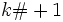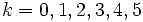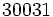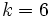Euclid prime

(diff) ← Older revision | Latest revision (diff) | Newer revision → (diff)
This article defines a property that can be evaluated for a prime number. In other words, every prime number either satisfies this property or does not satisfy this property.
View other properties of prime numbers | View other properties of natural numbers

Definition

A Euclid prime' is a prime number that is one more than a primorial. In other words, it is a prime number of the form, wheredenotes the product of the firstprimes.

A natural number of the formis termed a Euclid number, so a Euclid prime is a Euclid number that happens to be prime.

Occurrence

The ID of the sequence in the Online Encyclopedia of Integer Sequences is A018239

Initial values

The initial values of Euclid numbers are prime. For, the corresponding Euclid numbersare prime. The first Euclid number that is not prime is, corresponding to.